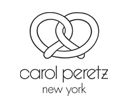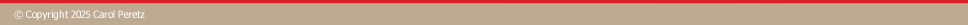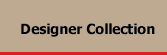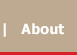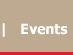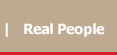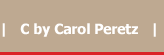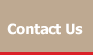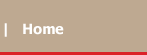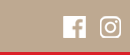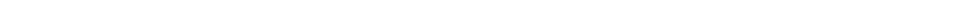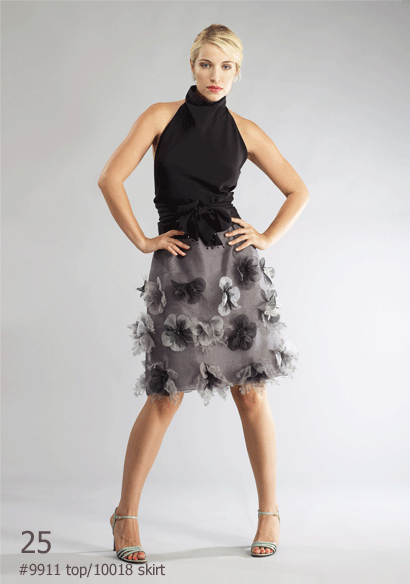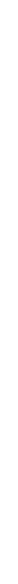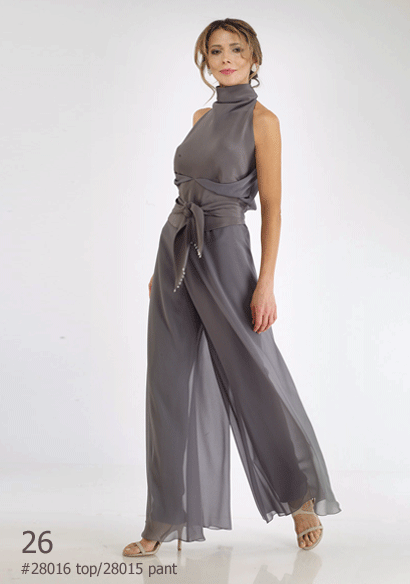Styles < Previous   Next > 1 and 2 3 and 4 5 and 6 7 and 8 9 and 10 11 and 12 13 and 14 15 and 16 17 and 18 19 and 20 21 and 22 23 and 24 25 and 26 27 and 28 29 and 30 31 and 32 33 and 34 35 and 36 37 and 38 39 and 40